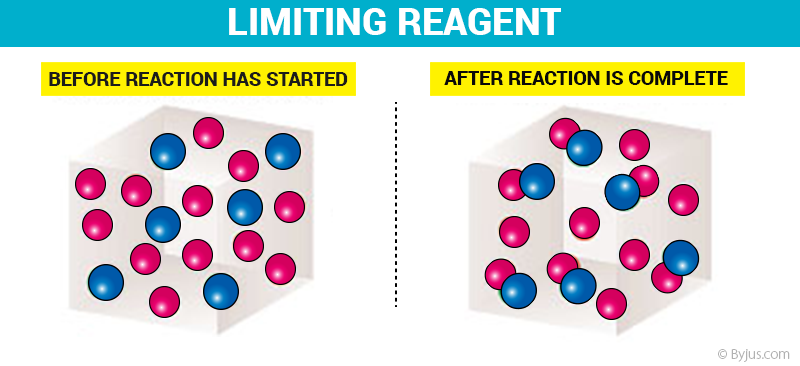# Limiting Reagent

## What is Limiting Reagents?

The reactant that is entirely used up in a reaction is called limiting reagent.

Limiting reagents are substances that are completely consumed in the completion of a chemical reaction. They are also referred to as limiting agents or limiting reactants. According to the stoichiometry of chemical reactions, a fixed amount of reactants is required for the completion of the reaction. Let us consider the following reaction of the formation of ammonia:

3H2 + N2 → 2NH3

In the reaction given above, 3 moles of Hydrogen gas are required to react with 1 mole of nitrogen gas to form 2 moles of ammonia. But what if, during the reaction, only 2 moles of hydrogen gas are available along with 1 mole of nitrogen.

In that case, the entire quantity of nitrogen cannot be used (because the entirety of nitrogen requires 3 moles of hydrogen gas to react). Hence, the hydrogen gas is limiting the reaction and is therefore called the limiting reagent for this reaction.

## Limiting Reagent Explanation

This reactant generally determines when the reaction will stop. The exact amount of reactant which will be needed to react with another element can be calculated from the reaction stoichiometry. The limiting reagent depends on the mole ratio, not on the masses of the reactants present.Limiting Reagent Before and After Reaction

From the illustration shown above, it can be observed that the limiting reactant is the reason the reaction cannot continue since there is nothing left to react with the excess reactant. It is the reactant that is entirely consumed over the course of the reaction.

## Limiting Reagent Examples

Consider 1 mol of oxygen and 1 mol of hydrogen are present to undergo the following reaction.

#### 2H2 + O2 → 2H2O

Since the reaction uses up hydrogen twice as fast as oxygen, the limiting reactant would be hydrogen.

Example: 100g of hydrochloric acid is added to 100g of zinc. Find the volume of hydrogen gas evolved under standard laboratory conditions.

Solution:

The chemical equation for these reactions is given below.

2HCl(aq) + Zn(s) → ZnCl2(aq) + H2(g)

Zinc chloride is formed in excess so the limiting reagent here is hydrochloric acid.

73g of HCl = 22.4l of H2
100g of HCl = yL of H2

y/22.4 = 100/73
y = (100 x 22.4)/73
y = 30.6L

Therefore, 33.6L of H2 is produced under standard laboratory conditions.

## How to find Limiting Reagent?

The determination of the limiting reactant is typically just a piece of a larger puzzle. In most limiting reactant stoichiometry problems, the real goal is to determine how much product could be formed from a particular reactant mixture. The limiting reactant or reagent can be determined by two methods.

1. Using the mole ration
2. Using the product approach

In order to calculate the mass of the product first, write the balanced equation and find out which reagent is in excess. Using the limiting reagent calculate the mass of the product.

The following points should be considered while attempting to identify the limiting reagent:

• When there are only two reactants, write the balanced chemical equation and check the amount of reactant B required to react with reactant A. When the amount of reactant B is greater, reactant A is the limiting reagent.
• The reactant which is in a lesser amount than is required by stoichiometry is the limiting reactant.
• In an alternate method of finding the limiting reagent, the amount of product formed by each reactant is calculated.
• The limiting reactant is the reactant from which the minimum amount of product is formed.
• Also, if we calculate the amount of one reactant needed to react with another reactant, then the reactant which is in shortage would be the required limiting reactant.

Thus, the required limiting reagent for the reaction can be identified using the points provided above. These reagents are very important while calculating the percentage yield of a given reaction.

## Applications Of Stoichiometry – Limiting Reagent## Frequently Asked Questions – FAQs

Q1

### What is the limiting reagent?

A reactant in a chemical reaction that determines the amount of product that is produced is the limiting reactant or limiting reagent. The reason for a limiting reactant is that elements and compounds react in a balanced chemical equation according to the mole ratio between them.

Q2

### What is the difference between reactant and reagent?

A reactant is a substance that reacts directly when the reaction is initiated, whereas a reagent is a substance that participates in a chemical reaction.

Q3

### Why is the limiting reactant important?

The limiting reagent/reactant is important because it can tell a chemist that only x moles of compounds can form when the perfect quantity is used with how much of this material they use, because it restricts the reaction, instead of the hypothetically.

Q4

### Are limiting reactants present in all reactions?

The limiting reactant determines the maximum amount of product that can be formed from the reactants when reactants are not present in stoichiometric quantities.

Q5

### What is the benefit of having a limiting reagent?

In a chemical reaction, the task of limiting reagent or reactant is significant because it can help the chemist predict the maximum quantity of reactant is consumed, since it restricts the reaction, only the necessary moles of products can be produced instead of the hypothetical yield where the perfect quantity is used.

Test your knowledge on Limiting Reagent